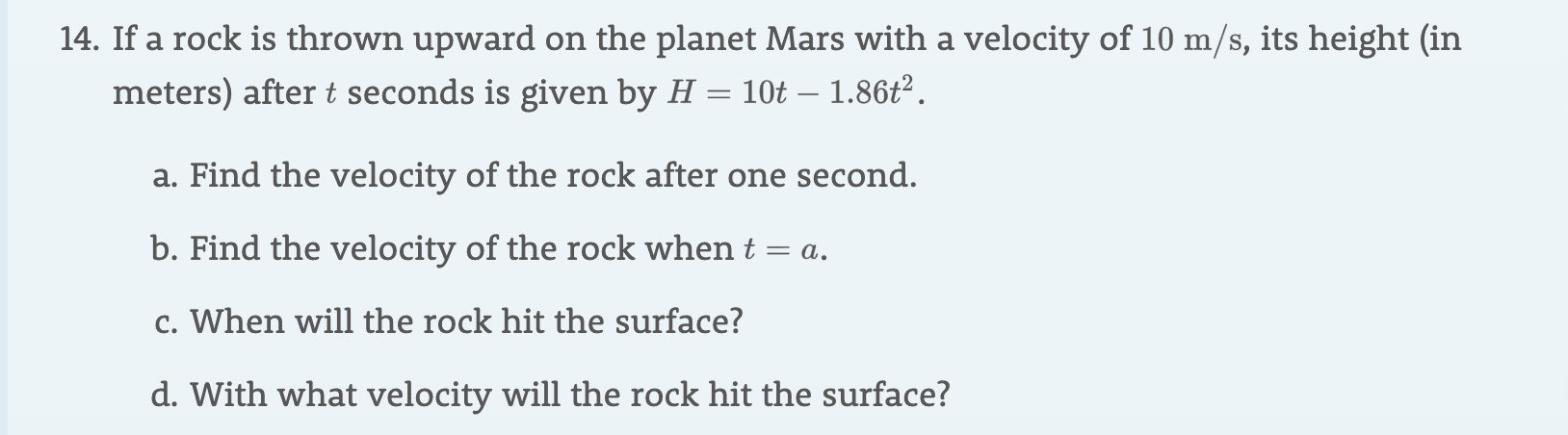# 14. If a rock is thrown upward on the planet Mars with a velocity of 10 m/s, its height (inmeters) after t seconds is given by H = 10t - 1.86t2a. Find the velocity of the rock after one second.b. Find the velocity of the rock when t = ac. When will the rock hit the surface?d. With what velocity will the rock hit the surface?

Question
26 viewshelp_outlineImage Transcriptionclose14. If a rock is thrown upward on the planet Mars with a velocity of 10 m/s, its height (in meters) after t seconds is given by H = 10t - 1.86t2 a. Find the velocity of the rock after one second. b. Find the velocity of the rock when t = a c. When will the rock hit the surface? d. With what velocity will the rock hit the surface? fullscreen
check_circle

Step 1

Since we only answer up to 3 sub-parts, we’ll answer the first 3. Please resubmit the question and specify the other subparts (up to 3) you’d like answered.

a. Velocity= first derivative of H

After 1 sec, we plug t=1.

v=10-(3.72(1))

v=6.28

Step 2

velocity of the rock when t=a, then H=10a-1.8...

### Want to see the full answer?

See Solution

#### Want to see this answer and more?

Solutions are written by subject experts who are available 24/7. Questions are typically answered within 1 hour.*

See Solution
*Response times may vary by subject and question.
Tagged in

### Other